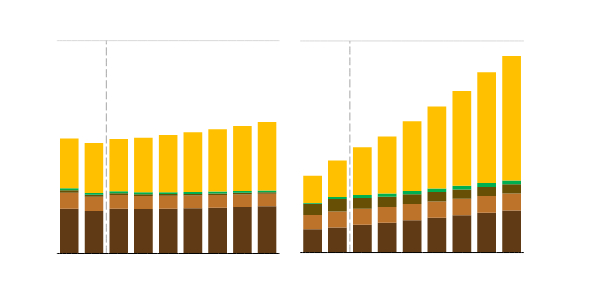# Pet Health care Quiz: Energy Generation And Energy Expenditure

25 Questions | Attempts: 488
ShareSettingsEnergy Generation and Energy Expenditure

• 1.
The best measurement of the short-term energy system activation by exercise is:
• A.

Blood glucose levels

• B.

Blood lactate levels

• C.

Blood pressure

• D.

Heart rate

• 2.
The fact that swim training for endurance does little to improve an athlete's distance running supports the principle of:
• A.

Generality

• B.

Individual differences

• C.

Reversibility

• D.

Specificity

• 3.
Factors that influence individual differences in their capacity for aerobic energy transfer include all of the following EXCEPT:
• A.

Mitochondrial content of the muscle

• B.

Personal motivation

• C.

Buffering of acid metabolites

• D.

Training intensity and duration

• 4.
All of the following are a type of test to determine VO2 max EXCEPT:
• A.

Cycle ergometer test

• B.

Step test

• C.

1_RM test

• D.

Walking test

• 5.
All of the following are types of open-circuit, indirect calorimetric procedures EXCEPT:
• A.

Bag technique

• B.

Portable spirometry

• C.

Bomb calorimeter

• D.

Computerized instrumentation

• 6.
Absolute values of VO2 max are expressed by:
• A.

L/min

• B.

ML/kg/min

• C.

Watts

• D.

M/min

• 7.
All of the following limit the accuracy of predicting VO2 max from submaximal exercise heart rate EXCEPT:
• A.

Linearity of the HR-VO2 relationship

• B.

Similar maximum heart rates for all subjects

• C.

Day-to-day variation in exercise heart rate

• D.

Assumed variability in exercise economy

• 8.
True or False? The capacity of the short-term energy system for a given muscle depends on its glycogen content:
• A.

True

• B.

False

• 9.
True or False? For adolescents and adults, power scores based on body mass (kg) are higher for females than for age-matched males.
• A.

True

• B.

False

• 10.
True or False?  When increasing the intensity of an ongoing exercise procedure fails to produce further increases in VO2, this suggests that VO2 max has been achieved.
• A.

True

• B.

False

• 11.
True or False?  Genetic factors have almost no impact on a person's VO2 max.
• A.

True

• B.

False

• 12.
True or False? In order to be a suitable and valid test, a performance test must be similar to the activity or sport for which energy capacity is evaluated.
• A.

True

• B.

False

• 13.
True or False? Pregnancy alone does not usually impede participation in aerobic exercise of moderate intensity.
• A.

True

• B.

False

• 14.
Three METs equals:
• A.

5.5 mL/kg/min

• B.

10.5 mL/kg/min

• C.

3.5 mL/kg/min

• D.

7 mL/kg/min

• 15.
Hand-held weights help runners to burn calories but may be especially risky if the runners suffer from:
• A.

Asthma

• B.

Diabetes

• C.

Hypertension

• D.

Hypothyroid

• 16.
This is NOT a major contributor to total energy expenditure:
• A.

Energy associated with physical activity

• B.

Resting metabolic rate

• C.

Restorative processes of sleep

• D.

Thermogenic influences of food consumption and processing

• 17.
The energy cost for running a 26.2-mile marathon, not including recovery, varies the most depending on:
• A.

The runner's aerobic fitness level

• B.

The runner's body mass

• C.

The runner's power

• D.

The cost is 2600 kCal irrespective of other choices

• 18.
The basal metabolic rate is NOT:
• A.

Dependent on age and sex

• B.

Significantly higher than the resting metabolic rate

• C.

Measured by determining oxygen uptake

• D.

Proportionate to fat-free body mass

• 19.
To calculate an individual's resting daily energy expenditure (RDEE), this value is not relevant:
• A.

Average sleep time

• B.

Age

• C.

Height

• D.

Wieght

• 20.
Resting metabolism is least dependent on:
• A.

Core body temperature

• B.

Fat-free body mass

• C.

Glycogen storage levels

• D.

Pregnancy

• 21.
The energy cost for traveling the same distance running as compared to swimming is roughly:
• A.

10%

• B.

25%

• C.

50%

• D.

250%

• 22.
The three factors that largely determine success in aerobic endurance performance include all of the following EXCEPT:
• A.

VO2 max

• B.

Neuromuscular specificity

• C.

The ability to sustain effort at a large percetage of VO2 max

• D.

The efficiency of energy use and/or movement economy

• 23.
In general, a 60-year-old woman will have a higher resting metabolic rate than a 25-year-old man.
• A.

True

• B.

False

• 24.
Changes in the level of physical activity represent the most profound influence on energy expenditure for most humans.
• A.

True

• B.

False

• 25.
BMR tends to decrease with chronological age:
• A.

True

• B.

FalseBack to top
×

Wait!
Here's an interesting quiz for you.